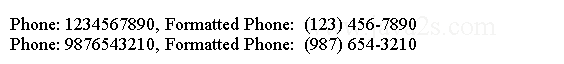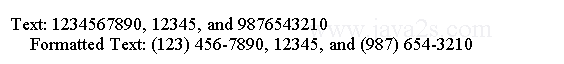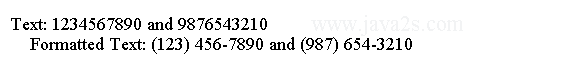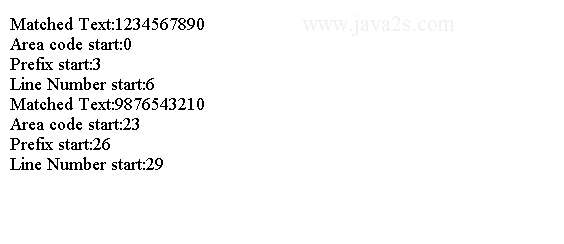# Java Regular Expression Tutorial - Java Regex Groups

We can group multiple characters as a unit by parentheses. For example, `(ab)`.

Each group in a regular expression has a group number, which starts at 1.

Method `groupCount()` from `Matcher` class returns the number of groups in the pattern associated with the Matcher instance.

The group 0 refers to the entire regular expression and is not reported by the groupCount() method.

Each left parenthesis inside a regular expression marks the start of a new group.

We can back reference group numbers in a regular expression.

Suppose we want to match text that starts with "abc" followed by "xyz", which is followed by "abc".

We can write a regular expression as "abcxyzabc".

We can use the back reference to rewrite the regular expression as "(abc)xyz\1". `\1` refers to group 1, which is `(abc)`.

`\2` to refer to group 2, `\3` to refer to group 3, and so on.

The following code shows how to display formatted phone numbers. In the regular expression `\b(\d{3})(\d{3})(\d{4})\b`, `\b` denotes that we are interested in matching ten digits only at word boundaries.

```import java.util.regex.Matcher;
import java.util.regex.Pattern;
// w ww.ja  v a2s  .  co m
public class Main {
public static void main(String[] args) {
String regex = "\\b(\\d{3})(\\d{3})(\\d{4})\\b";

Pattern p = Pattern.compile(regex);
String source = "1234567890, 12345,  and  9876543210";

Matcher m = p.matcher(source);

while (m.find()) {
System.out.println("Phone: " + m.group() + ", Formatted Phone:  ("
+ m.group(1) + ") " + m.group(2) + "-" + m.group(3));
}
}
}
```

The code above generates the following result.## Example

The following code shows how to reference groups in a replacement text.

`\$n`, where `n` is a group number, inside a replacement text refers to the matched text for group `n`.

For example, `\$1` refers to the first matched group. To reformat phone numbers, we would use `(\$1) \$2-\$3`.

```import java.util.regex.Matcher;
import java.util.regex.Pattern;
/*ww  w .j a  v  a 2  s  . co m*/
public class Main {
public static void main(String[] args) {
String regex = "\\b(\\d{3})(\\d{3})(\\d{4})\\b";
String replacementText = "(\$1) \$2-\$3";
String source = "1234567890, 12345, and 9876543210";

Pattern p = Pattern.compile(regex);
Matcher m = p.matcher(source);

String formattedSource = m.replaceAll(replacementText);

System.out.println("Text: " + source);
System.out.println("Formatted Text: " + formattedSource);
}
}
```

The code above generates the following result.## Named Groups

We can use named groups in regular expressions.

We can name a group then back reference groups using their names.

We can reference group names in replacement text and get the matched text using the group names.

The format to define a named group is

```(?<groupName>pattern)
```

A pair of parentheses marks a group. The start parenthesis is followed by a `?` and a group name placed in angle brackets.

The group name can only have letters and digits, and can only start with a letter.

The following regular expression has three named groups.

• areaCode
• prefix
• postPhoneNumber

The regular expression matches a 10-digit phone number.

```\b(?<areaCode>\d{3})(?<prefix>\d{3})(?<postPhoneNumber>\d{4})\b
```

The following code shows how to use the named group.

```String  replacementText = "(\${areaCode}) \${prefix}-\${postPhoneNumber}";
```

We can mix the group number and a group name.

The above regular expression can be rewritten as follows.

```String  replacementText = "(\${areaCode}) \${prefix}-\$3";
```

The following code shows how to use group names in a regular expression and how to use the names in a replacement text.

```import java.util.regex.Matcher;
import java.util.regex.Pattern;
// w  ww . j a  v  a  2 s. c o m
public class Main {
public static void main(String[] args) {
String regex = "\\b(?<areaCode>\\d{3})(?<prefix>\\d{3})(?<postPhoneNumber>\\d{4})\\b";

String replacementText = "(\${areaCode}) \${prefix}-\$3";
String source = "1234567890 and 9876543210";
Pattern p = Pattern.compile(regex);

Matcher m = p.matcher(source);

String formattedSource = m.replaceAll(replacementText);

System.out.println("Text: " + source);
System.out.println("Formatted Text: " + formattedSource);
}
}
```

The code above generates the following result.## Group boundary

We can use `start()` and `end()` methods to get the match boundary for groups. These methods are overloaded:

```int start()
int start(int groupNumber)
int start(String groupName)
int end()
int end(int groupNumber)
int  end(String groupName)
```

The methods return the start and end of the previous match.

The following code shows how to match 10-digit phone number and print the start of each group for each successful match.

```import java.util.regex.Matcher;
import java.util.regex.Pattern;
//w  ww  .j  av  a  2s.  c o m
public class Main {
public static void main(String[] args) {
String regex = "\\b(?<areaCode>\\d{3})(?<prefix>\\d{3})(?<postPhoneNumber>\\d{4})\\b";
String source = "1234567890, 12345, and 9876543210";
Pattern p = Pattern.compile(regex);

Matcher m = p.matcher(source);
while (m.find()) {
String matchedText = m.group();
int start1 = m.start("areaCode");
int start2 = m.start("prefix");
int start3 = m.start("postPhoneNumber");
System.out.println("Matched Text:" + matchedText);
System.out.println("Area code start:" + start1);
System.out.println("Prefix start:" + start2);
System.out.println("Line Number start:" + start3);
}
}
}
```

The code above generates the following result.